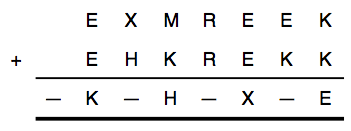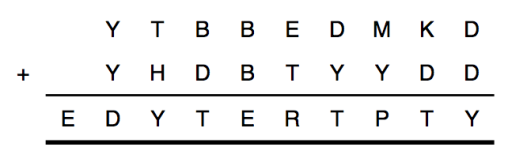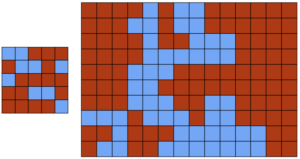## Polygons with perimeter and vertex budgets

his week’s Riddler Classic involves designing maximum-area polygons with a fixed budget on the length of the perimeter and the number of vertices. The original problem involved designing enclosures for hamsters, but I have paraphrased the problem to make it more concise.

You want to build a polygonal enclosure consisting of posts connected by walls. Each post weighs $k$ kg. The walls weigh $1$ kg per meter. You are allowed a maximum budget of $1$ kg for the posts and walls.

What’s the greatest value of $k$ for which you should use four posts rather than three?

Extra credit: For which values of $k$ should you use five posts, six posts, seven posts, and so on?

Here is my solution:
[Show Solution]

## Settlers in a circle

In this Riddler problem, the goal is to spread out settlements in a circle so that they are as far apart as possible:

Antisocial settlers are building houses on a prairie that’s a perfect circle with a radius of 1 mile. Each settler wants to live as far apart from his or her nearest neighbor as possible. To accomplish that, the settlers will overcome their antisocial behavior and work together so that the average distance between each settler and his or her nearest neighbor is as large as possible.

At first, there were slated to be seven settlers. Arranging that was easy enough: One will build his house in the center of the circle, while the other six will form a regular hexagon along its circumference. Every settler will be exactly 1 mile from his nearest neighbor, so the average distance is 1 mile.

However, at the last minute, one settler cancels his move to the prairie altogether (he’s really antisocial). That leaves six settlers. Does that mean the settlers can live further away from each other than they would have if there were seven settlers? Where will the six settlers ultimately build their houses, and what’s the maximum average distance between nearest neighbors?

Here is my solution:
[Show Solution]

## Alice and Bob fall in love

In this interesting Riddler problem, we’re dealing with a possibly unbounded sequence of… children? Here it goes:

As you may know, having one child, let alone many, is a lot of work. But Alice and Bob realized children require less of their parents’ time as they grow older. They figured out that the work involved in having a child equals one divided by the age of the child in years. (Yes, that means the work is infinite for a child right after they are born. That may be true.)

Anyhow, since having a new child is a lot of work, Alice and Bob don’t want to have another child until the total work required by all their other children is 1 or less. Suppose they have their first child at time T=0. When T=1, their only child is turns 1, so the work involved is 1, and so they have their second child. After roughly another 1.61 years, their children are roughly 1.61 and 2.61, the work required has dropped back down to 1, and so they have their third child. And so on.

(Feel free to ignore twins, deaths, the real-world inability to decide exactly when you have a child, and so on.)

Five questions: Does it make sense for Alice and Bob to have an infinite number of children? Does the time between kids increase as they have more and more kids? What can we say about when they have their Nth child — can we predict it with a formula? Does the size of their brood over time show asymptotic behavior? If so, what are its bounds?

Here is an explanation of my derivation:
[Show Solution]

If you’re just interested in the answers to the questions, here they are:
[Show Solution]

## Smudged secret messages

This Riddler is a twist on a classic problem: decoding equations! Here is the paraphrased problem:

The goal is to decode two equations. In each of them, every different letter stands for a different digit. But there is a minor problem in both equations. In the first equation, letters accidentally were smudged and are now unreadable. (These are represented with dashes below.) But we know that all 10 digits, 0 through 9, appear in the equation.What digits belong to what letters, and what are the dashes? In the second equation, one of the letters in the equation is wrong. But we don’t know which one. Which is it?Here is my detailed solution:
[Show Solution]

If you’re just interested in the answers, here they are:
[Show Solution]

Consider four towns arranged to form the corners of a square, where each side is 10 miles long. You own a road-building company. The state has offered you \$28 million to construct a road system linking all four towns in some way, and it costs you \$1 million to build one mile of road. Can you turn a profit if you take the job?

Extra credit: How does your business calculus change if there were five towns arranged as a pentagon? Six as a hexagon? Etc.?

Here is a longer explanation:
[Show Solution]

Here is the solution with minimal explanation:
[Show Solution]

## Ostomachion coloring

The following problems appeared in The Riddler. And it features an interesting combination of an ancient game with the four-color theorem.

The famous four-color theorem states, essentially, that you can color in the regions of any map using at most four colors in such a way that no neighboring regions share a color. A computer-based proof of the theorem was offered in 1976.

Some 2,200 years earlier, the legendary Greek mathematician Archimedes described something called an Ostomachion (shown below). It’s a group of pieces, similar to tangrams, that divides a 12-by-12 square into 14 regions. The object is to rearrange the pieces into interesting shapes, such as a Tyrannosaurus rex. It’s often called the oldest known mathematical puzzle.

Your challenge today: Color in the regions of the Ostomachion square with four colors such that each color shades an equal area. (That is, each color needs to shade 36 square units.) The coloring must also satisfy the constraint that no adjacent regions are the same color.

Extra credit: How many solutions to this challenge are there?Here are the details of how I solved the problem:
[Show Solution]

To jump straight to the solution (and pretty pictures!) see below.
[Show Solution]

## Cracking the safe

The following problem appeared in The Riddler and it’s about finding the right code sequence to crack open a safe.

A safe has three locks, each of which is unlocked by a card, like a hotel room door. Each lock (call them 1, 2 and 3) and can be opened using one of three key cards (A, B or C). To open the safe, each of the cards must be inserted into a lock slot and then someone must press a button labeled “Attempt To Open.”

The locks function independently. If the correct key card is inserted into a lock when the button is pressed, that lock will change state — going from locked to unlocked or unlocked to locked. If an incorrect key card is inserted in a lock when the attempt button is pressed, nothing happens — that lock will either remain locked or remain unlocked. The safe will open when all three locks are unlocked. Other than the safe opening, there is no way to know whether one, two or all three of the locks are locked.

Your job as master safecracker is to open the locked safe as efficiently as possible. What is the minimum number of button-press attempts that will guarantee that the safe opens, and what sequence of attempts should you use?

Here is my solution.
[Show Solution]

## Squaring the square

This Riddler puzzle is about tiling a square using smaller squares.

You are handed a piece of paper containing the 13-by-13 square shown below, and you must divide it into some smaller square pieces. If you are only allowed to cut along the lines, what is the smallest number of squares you can divide this larger square into? (You could, for example, divide it into one 12-by-12 square and 25 one-by-one squares for a total of 26 squares, but you can do much better.)Here is how I solved the problem:
[Show Solution]

And here is the tl;dr, just the solutions!
[Show Solution]

## Timing a stoplight just right

This Riddler is about how to perfectly time a stoplight, something we’ve all had to deal with!

You are driving your car on a perfectly flat, straight road. You are the only one on the road and you can see anything ahead of you perfectly. At time t=0, you are at Point A, cruising along at a speed of 100 kilometers per hour, which is the speed limit for the whole road. You want to reach Point C, exactly 4 kilometers ahead, in the shortest time possible. But, at Point B, 2 kilometers ahead of you, there is a traffic light.

At time t=0, the light is green, but you don’t know how long it has been green. You do know that at the beginning of each second, there is a 1 percent chance that the light will turn yellow. Once it turns yellow, it remains yellow for 5 seconds and then turns red for 20 seconds. Your car can accelerate or decelerate at a maximum rate of 2 meters per second-squared. You must always drive at or below the speed limit. You can pass through the intersection when the traffic light is yellow, but not when it is red.

What is the best strategy to reach your destination as soon as possible?

Here is my solution:
[Show Solution]

## Rig the election with math!

This Riddler problem is about gerrymandering. How to redraw borders to sway a vote one way or another.

Below is a rough approximation of Colorado’s voter preferences, based on county-level results from the 2012 presidential election, in a 14-by-10 grid. Colorado has seven districts, so each would have 20 voters in this model. In each district, the party with the most votes will win. The districts must be non-overlapping and contiguous (that is, each square in a district must share an edge with at least one other square in the district). What is the most districts that the Red Party could win? What about the Blue Party? (Assume ties within a district are considered wins for the party of your choice.)

Two boards are provided, a test 5×5 grid and the larger 14×10 grid:Here is a first solution, using some simple logic and intuition:
[Show Solution]

And here is a computational approach, using integer programming:
[Show Solution]